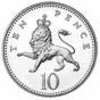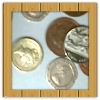#### You may also like### Coin Tossing Games

You and I play a game involving successive throws of a fair coin. Suppose I pick HH and you pick TH. The coin is thrown repeatedly until we see either two heads in a row (I win) or a tail followed by a head (you win). What is the probability that you win?### Win or Lose?

A gambler bets half the money in his pocket on the toss of a coin, winning an equal amount for a head and losing his money if the result is a tail. After 2n plays he has won exactly n times. Has he more money than he started with?A counter is placed in the bottom right hand corner of a grid. You toss a coin and move the star according to the following rules: ... What is the probability that you end up in the top left-hand corner of the grid?

# Same Number!

##### Age 14 to 16 Challenge Level:

Joseph from Wilson's School gave a comprehensive answer to the problem.

I think it is likely for everyone's number to be different as there are 225 numbers and only 30 people. There are only a few times when everyone's numbers are different.
Yes, I am surprised by the fact that everyone's number was different only a few times.

Rajeev from Haberdashers' Aske's Boys' School gave an excellent answer to the problem

In a class of 30 if a teacher asks the class to write down a number from 1 to 225 then the probabilitity of same number being read out is as follows:
The probability of the first two students both reading out different numbers is $\frac{224}{225}$
The chance of a 3rd student reading out a different number is $\frac{223}{225}$.
The probability that the first 3 students will all read out different numbers is $\frac{224}{225}\times\frac{ 223}{225}$
The chance of a 4th student reading out a different number is $\frac{222}{225}$.
The probability that the first 4 students will all read out different numbers is $\frac{224}{225}\times\frac{ 223}{225}\times\frac{ 222}{225}$
The probability that the whole class will all read out different numbers is $\frac{224}{225}\times\frac{ 223}{225}\times\frac{ 222}{225}.......\times\frac{196}{225}$.
This implies the probability that at least two students have written the same number is $1 - \frac{224}{225}\times\frac{ 223}{225}\times\frac{ 222}{225}.......\times\frac{196}{225}$

With regard to the problem of how many people you need in a room so that the chance of there being two people with the same birthday greater than 50% is to think that if two people came in a room and they did not share a birthday then the probability is $\frac{364}{365}$.
With three people not sharing the same birthday it is $\frac{364}{365}\times\frac{363}{365}$.
So if you keep multiplying like this you will eventually find an answer which is just less than 0.50 and that is $\frac{364}{365}\times\frac{363}{365}.....\times\frac{343}{365}$ and that works out at 0.49.
That means 23 people as $365-343+1=23$. So the probability that at least two people share the same birthday is $1- 0.49=0.51$.

A student presented a very clear elucidation of the calculation process above:

Start with just one person (a). they can choose any number between 225 without any overlaps obviously as they are the first person.The probability of no overlaps is therefore $\frac{225}{225}=1$
If we model this with two people, the probability of person b NOT getting the same number as person a is $\frac{224}{225}$
Introducing a third person (c), the probability of them ALSO sharing a number with neither a nor b is $\frac{224}{225}\times\frac{223}{225}$ (because there are now only 223 possibilities for c without number-sharing). Intoducing a fourth person (d), the probability that all will have different numbers is $1\times\frac{224}{225}\times\frac{223}{225}\times\frac{222}{225}$...and so on
The probability of two or more people sharing numbers in a given population of x, where n is the maximum number, is $1-\frac{n(n-1)(n-2)....(n-(x-1))(n-x)}{n^x}=P$ (Probability of match) knowing two of n, x, or P means we can feed in the numbers to find the last variable.

Well done to everyone!